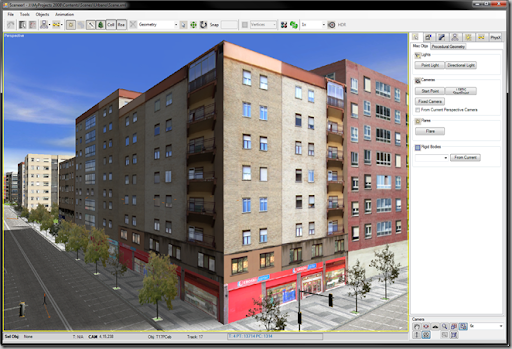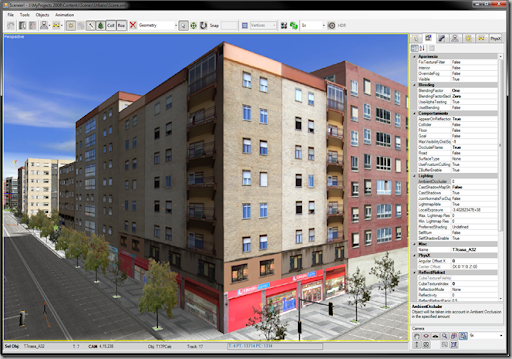## Monday, 17 September 2012

### Properly calculating the diffuse contribution of lights in HLSL Shaders

It’s been many years since Vertex and Pixel Shaders came out, and several years too since the Fixed Pipeline is deprecated, but there are still many questions in the forums out there asking about how to properly calculate the diffuse contribution of Lights. This paper has a great tutorial about the issue, and includes a whole Shader that mimics the Fixed Pipeline behavior. However, we will see here how to perform just the basic calculations, just in case you don’t need to emulate the full pipeline.
First thing is to write some D3D9 code that allows you to switch from the old Fixed Pipeline and your own Shaders, using the same parameters. Doing so, you will easily find any behavior differences in light calculations. You can read more about how D3D9 Fixed Pipeline calculates lighting in this page.
When writing shaders, people tend to calculate the diffuse contribution like:
Out.Color = (materialAmbient * lightAmbient) + (materialDiffuse * lightDiffuse * dot(Normal, L));
Where L is the vector from the vertex position (in world coordinates) to the light.
Apart from not doing any specular or emissive calculations (which could not be necessary in many cases, depending on your scenario), there are several mistakes in that approach:
1.- You don’t want the dot to return negative values, because it will black out colors wrongly. So, you need to clamp it to the 0..1 range, using the saturate operator: saturate(dot(Normal, L))
2.- In order to get the same results as the Fixed Pipeline, you should include Attenuation calculations, because they modify the intensity of light with the distance between the point being lit and the light source. Attenuation (as opposed to what its name suggests), not only attenuates light, but also can increase intensity in some circumstances. (See below how to properly calculate attenuation factors)
3.- Once you are calculating attenuation, you should remove the materialDiffuse factor from the previous equation, as you don’t want it to be attenuated too. You will apply it later, when the entire lighting contribution is properly calculated and attenuated.
Keeping those 3 things in mind, the final calculation in a vertex shader would be:
float4 LightContrib = (0.f, 0.f, 0.f, 0.f);
float fAtten = 1.f;

// 1.- First, we store the total ambient light in the scene (multiplication of material_ambient, light_ambient, and any other global ambient component)
Out.Color = mMaterialAmbient * mLightAmbient;

// 2.- Calculate vector from point to Light (both normalized and not-normalized versions, as we might need to calculate its length later)
float pointToLightDif = mLightPos - P;
float3 pointToLightNormalized = normalize(pointToLightDif);

// 3.- Calculate dot product between world_normal and pointToLightNormalized
float NDotL = dot(Nw, pointToLightNormalized);
if(NDotL > 0)
{
LightContrib = mLightDiffuse * NDotL * mLightDivider;

float LD = length(pointToLightDif);
if(LD > mLightRange)
fAtten = 0.f;
else
fAtten = 1.f/(mLightAtt0 + mLightAtt1*LD + mLightAtt2*LD*LD);

LightContrib *= fAtten;
}
Out.Color += LightContrib * mMaterialColor;
Out.Color = saturate(Out.Color);

### Comparison

First image is the Programmable version. You can slightly tell it by the reflections on the windows.Second image is the Fixed Pipeline version (no real time reflections on windows):